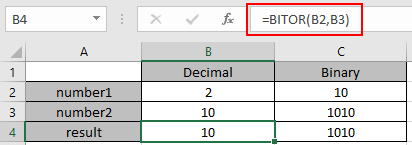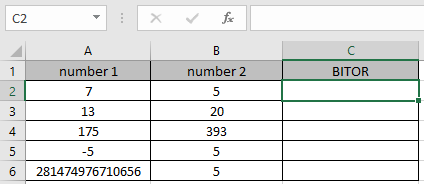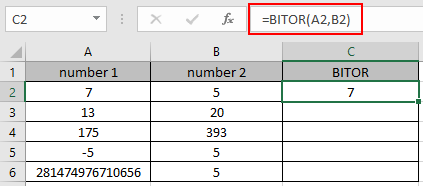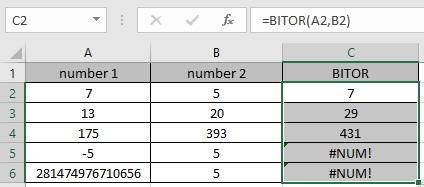# How to use the BITOR Function in Excel

BITOR function in excel perform the Bitwise OR operation between the two decimal numbers and returns the decimal number after the operation performed.
OR operation is a logical operator used in communication processes. OR operator is performed over the 2 bits ( 0 or 1 ). The operation takes place like in table shown below.

 Bit 1 Bit 2 OR operation 0 0 0 0 1 1 1 0 1 1 1 1

Here the decimal numbers be changed in binary and the OR operation be performed over each bit with the corresponding bits.

 Decimal Binary number1 2 010 number2 4 100 result 6 110

The different highlighted bits is to show how the Bitwise OR operation occurs between the two numbers. Use the DEC2BIN function ( decimal to binary conversion) & BIN2DEC function ( binary to decimal conversion) to understand BITOR function’s result step by step in Excel.

The BITOR function returns a bitwise 'OR' of two numbers.
Syntax:

=BITOR ( number1, number2)

number1 : a positive integer number of base 10 (decimal number).
number2 : a positive integer number of base 10 (decimal number).

Here is the excel representation of the functionNow let’s get more understanding of the function via using them in some examples.Here we have some values as number1 & number 2 in Columns. We need to get the Bitwise 'OR’ operation on the given numbers

Use the formula :

=BITOR (A2, B2)

A2 : number provided to the function as cell reference
B2 : number provided to the function as cell referenceThe Bitwise 'OR’ operation on 7 ( 111 ) & 5 ( 101 ). Resulting binary number would be 101. So the decimal number representing 101 is 5.

Now copy the formula to other cells using the Ctrl + D shortcut key.As you can see here the BITOR function returns the result of the input values.

Notes:

1. Numbers can be given as argument to the function directly or using the cell reference in excel.
2. The numbers must be a valid decimal number between 0 & 281474976710655.
3. The function returns the #NUM! Error, If either of the decimal number is less than 0 or greater than 281474976710655.
4. The function returns the #VALUE! Error, If the input number is text or non-numeric.

Hope you understood how to use BITOR function and referring cell in Excel. Explore more articles on Excel mathematical conversion functions here. Please feel free to state your query or feedback for the above article.

Terms and Conditions of use

The applications/code on this site are distributed as is and without warranties or liability. In no event shall the owner of the copyrights, or the authors of the applications/code be liable for any loss of profit, any problems or any damage resulting from the use or evaluation of the applications/code.